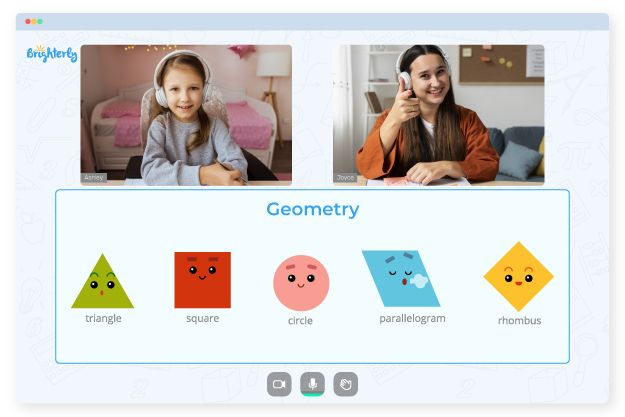Geometry is one of the first parts of mathematics students learn. Math worksheets help them go through this stage, allowing for detailed knowledge of the subject while also being useful during preparation for exams. Therefore, finding area 3rd grade worksheets and giving them to your child are the necessary steps parents should take.

## What Is a 3rd Grade Area Worksheet?

Area worksheets for 3rd-grade students mainly contain questions and activities about calculating the area of ​​different geometrical shapes, including triangles, rectangles, and squares. 3rd-grade area worksheets introduce students to figures such as rectangles and triangles and teach them how to calculate those figures’ size. Once students are familiar with the basic concepts of geometry and can successfully solve problems, they can move on to worksheets where they need to calculate areas of rhombuses and other complex shapes.

Math for Kids

Is Your Child Struggling With Math?
1:1 Online Math TutoringSince geometry is a broad course, students find it challenging to gain a complete insight into all ideas. Thus, area worksheets grade 3 students use come in handy at that point. They often contain questions and problems that help students understand the importance and real-world application of geometry concepts.

## What Area 3rd Grade Worksheets Focuses On

• Area and perimeter for rectangles and squares, as well as standard grids and images
• Fun world problems, such as having students calculate the length of the missing side given an area or perimeter
• A complex rectangular problem that students can solve as they progress to higher learning levels

## Benefits of 3rd Grade Area Worksheets

Worksheets in each discipline are specially designed to help students learn at their own pace. They also tend to include interactive images that make the topic easy, understandable, and fun.### Area Worksheets 3rd Grade PDF### Area Worksheets 3rd Grade PDF### Area Worksheets 3rd Grade PDF### Area Worksheets 3rd Grade PDF

Grade 3 math exercises help students understand the area concept and visualize related geometric theorems.

These worksheets start with simple shapes, helping students understand the application of various formulas. Gradually, the levels begin to advance, and students solve more complex problems that involve working with mathematical formulas.

### Area and Perimeter Worksheets

Struggling with Geometry?• Is your child having trouble understanding the concept of geometry?
• An online tutor could be of assistance.

Does your child need extra help with mastering geometry? Try studying with an online tutor.